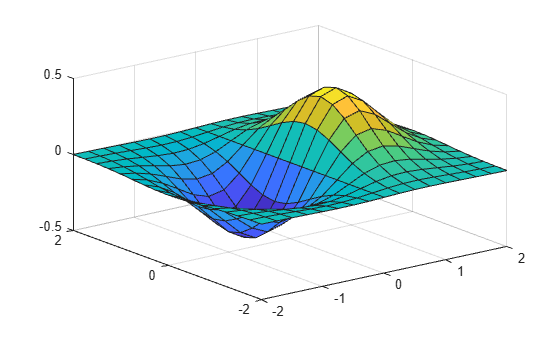# meshgrid

2-D and 3-D grids

## Syntax

``````[X,Y] = meshgrid(x,y)``````
``````[X,Y] = meshgrid(x)``````
``````[X,Y,Z] = meshgrid(x,y,z)``````
``````[X,Y,Z] = meshgrid(x)``````

## Description

example

``````[X,Y] = meshgrid(x,y)``` returns 2-D grid coordinates based on the coordinates contained in vectors `x` and `y`. `X` is a matrix where each row is a copy of `x`, and `Y` is a matrix where each column is a copy of `y`. The grid represented by the coordinates `X` and `Y` has `length(y)` rows and `length(x)` columns.```

example

``````[X,Y] = meshgrid(x)``` is the same as ```[X,Y] = meshgrid(x,x)```, returning square grid coordinates with grid size `length(x)`-by-`length(x)`.```

example

``````[X,Y,Z] = meshgrid(x,y,z)``` returns 3-D grid coordinates defined by the vectors `x`, `y`, and `z`. The grid represented by `X`, `Y`, and `Z` has size `length(y)`-by-`length(x)`-by-`length(z)`.```

example

``````[X,Y,Z] = meshgrid(x)``` is the same as ```[X,Y,Z] = meshgrid(x,x,x)```, returning 3-D grid coordinates with grid size `length(x)`-by-`length(x)`-by-`length(x)`.```

## Examples

collapse all

Create 2-D grid coordinates with x-coordinates defined by the vector `x` and y-coordinates defined by the vector `y`.

```x = 1:3; y = 1:5; [X,Y] = meshgrid(x,y)```
```X = 5×3 1 2 3 1 2 3 1 2 3 1 2 3 1 2 3 ```
```Y = 5×3 1 1 1 2 2 2 3 3 3 4 4 4 5 5 5 ```

Evaluate the expression ${x}^{2}+{y}^{2}$ over the 2-D grid.

`X.^2 + Y.^2`
```ans = 5×3 2 5 10 5 8 13 10 13 18 17 20 25 26 29 34 ```

Create a 2-D grid with uniformly spaced x-coordinates and y-coordinates in the interval [-2,2].

```x = -2:0.25:2; y = x; [X,Y] = meshgrid(x);```

Evaluate and plot the function $f\left(x,y\right)=x{e}^{-{x}^{2}-{y}^{2}}$ over the 2-D grid.

```F = X.*exp(-X.^2-Y.^2); surf(X,Y,F)```Starting in R2016b, it is not always necessary to create the grid before operating over it. For example, computing the expression $x{e}^{-{x}^{2}-{y}^{2}}$ implicitly expands the vectors `x` and `y`. For more information on implicit expansion, see Array vs. Matrix Operations.

`surf(x,y,x.*exp(-x.^2-(y').^2))`Create 3-D grid coordinates from x-, y-, and z-coordinates defined in the interval [0,6], and evaluate the expression ${x}^{2}+{y}^{2}+{z}^{2}$.

```x = 0:2:6; y = 0:1:6; z = 0:3:6; [X,Y,Z] = meshgrid(x,y,z); F = X.^2 + Y.^2 + Z.^2;```

Determine the size of the grid. The three coordinate vectors have different lengths, forming a rectangular box of grid points.

`gridsize = size(F)`
```gridsize = 1×3 7 4 3 ```

Use the single-input syntax to generate a uniformly spaced 3-D grid based on the coordinates defined in `x`. The new grid forms a cube of grid points.

```[X,Y,Z] = meshgrid(x); G = X.^2 + Y.^2 + Z.^2; gridsize = size(G)```
```gridsize = 1×3 4 4 4 ```

## Input Arguments

collapse all

x-coordinates of points, specified as a vector.

Data Types: `single` | `double` | `int8` | `int16` | `int32` | `int64` | `uint8` | `uint16` | `uint32` | `uint64`

y-coordinates of points, specified as a vector.

Data Types: `single` | `double` | `int8` | `int16` | `int32` | `int64` | `uint8` | `uint16` | `uint32` | `uint64`

z-coordinates of points, specified as a vector.

Data Types: `single` | `double` | `int8` | `int16` | `int32` | `int64` | `uint8` | `uint16` | `uint32` | `uint64`

## Output Arguments

collapse all

x-coordinates over a grid, returned as a 2-D (two inputs) or 3-D array (three inputs).

y-coordinates over a grid, returned as a 2-D (two inputs) or 3-D array (three inputs).

z-coordinates over a grid, returned as a 3-D array.